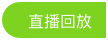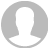﻿ 龙星计划《机器学习》_Moore8摩尔吧## 龙星计划《机器学习》

2017/10/23 10:30

82 人已报名

﻿ 直播回看
• 课程概述
• 学员评价
• 课程问答
• 附件下载

lecture 1: Introduction to ML and review of linear algebra, probability, statistics (kai)

lecture 2: linear model (tong)

lecture 3: overfitting and regularization (tong)

lecture 4: linear classification (kai)

lecture 5: basis expansion and kernel methods (kai)

lecture 6: model selection and evaluation (kai)

lecture 7: model combination (tong)

lecture 8: boosting and bagging (tong)

lecture 9: overview of learning theory (tong)

lecture 10: optimization in machine learning (tong)

lecture 11: online learning (tong)

lecture 12: sparsity models (tong)

lecture 13: introduction to graphical models (kai)

lecture 14: structured learning (kai)

lecture 15: feature learning and deep learning (kai)

lecture 16: transfer learning and semi supervised learning (kai)

lecture 17: matrix factorization and recommendations (kai)

lecture 18: learning on images (kai)

lecture 19: learning on the web (tong)